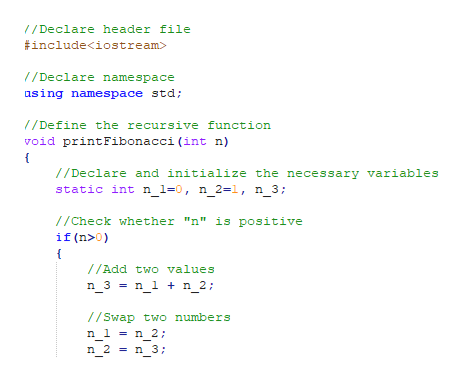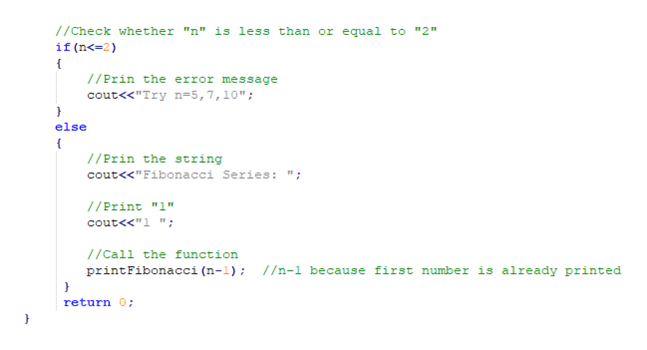# Write a C++ program:to iteratively compute Fibonacci numbers (named after an Italian mathematician). Some Fibonacci numbers are 1,1,2,3,5,8,... where the next number is found by summing the preceding two numbers. Your program is not assured of recieving good data, thus if -2 is recieved as the number of numbers, then an error should be reported. Try n = 5, 7, 10, -3

Question
31 views

Write a C++ program:

to iteratively compute Fibonacci numbers (named after an Italian mathematician). Some Fibonacci numbers are 1,1,2,3,5,8,... where the next number is found by summing the preceding two numbers. Your program is not assured of recieving good data, thus if -2 is recieved as the number of numbers, then an error should be reported.

Try n = 5, 7, 10, -3

check_circle

star
star
star
star
star
1 Rating
Step 1

A C++ program to generate fibonacci series using recursion is given below,

Program:

#include<iostream>

//Declare namespace

using namespace std;

//Define the recursive function

void print_Fibonacci(int n)

{

//Declare ans initialize the necessary variables

static int n_1=0, n_2=1, n_3;

//Check whether "n" is positive

if(n>0)

{

n_3 = n_1 + n_2;

//Swap two numbers

n_1 = n_2;

n_2 = n_3;

//Print the calculated value

cout<<n_3<<" ";

//Call the function iteratively

print_Fibonacci(n-1);

}

}

//Define the main() function

int main()

{

//Declare the variable

int n;

//Prompt the user for the input

cout<<"Enter the number of elements: ";

//Get the user input

cin>>n;

//Check whether "n" is less than or equal to "2"

if(n<=2)

{

//Prin the error message

cout<<"Try n=5,7,10";

}

else

{

//Prin the string

cout<<"Fibonacci Series: ";

//Print "1"

cout<<"1 ";

//Call the function

print_Fibonacci(n-1);  //n-1 because first number is already printed

}

return 0;

}

Screenshot of program #1:help_outlineImage Transcriptionclose1/Declare header file #include /Declare namespace asing namespace std; /Define the recursive function void printFibonacci(int n) //Declare and initialize the necessary variables static int n_1=0, n_2=1, n_3; //Check whether "n" is positive if (n>0) //Add two values n_3 = n_1 n_2: 1/Swap two numbers n 1 n 2; n 3 n_2 fullscreen
Step 2

Screenshot of program #2:

Step 3

Screenshot of program #3...help_outlineImage Transcriptionclose//Check whether "n" is less than or equal to "2" if (n<-2) //Prin the error message cout<<"Try n=5,7, 10"; else { //Prin the string cout<<"Fibonacci Series: /Print "1" cout<<"1 "; /Call the function printFibonacci (n-l); //n-1 because first number is already printed return 0 fullscreen

### Want to see the full answer?

See Solution

#### Want to see this answer and more?

Solutions are written by subject experts who are available 24/7. Questions are typically answered within 1 hour.*

See Solution
*Response times may vary by subject and question.
Tagged in

### Computer Science